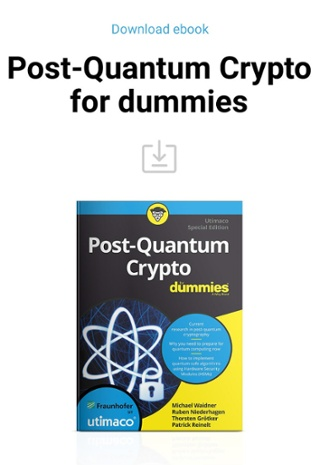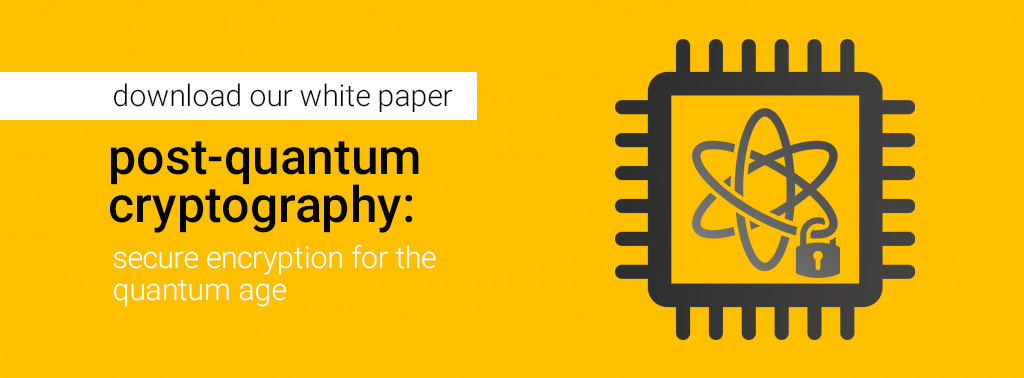•written on Feb 14, 2019
by Utimaco

### NIST shortlisted 26 algorithms for focal post-quantum research - get all the details here

post-quantum research network

It has taken over two decades to establish and secure the current e-commerce applications. The security of most of these systems principally relies on cryptographic algorithms which have served the purpose till now. Since the initiation and evolution of quantum computing, some cryptographic algorithms have threats. To mitigate the security gap, numerous post-quantum algorithms have been proposed. This article enlightens the journey towards post-quantum algorithms and security parameters of the newly proposed post-quantum algorithms.

## Current crypto implementations and application

The process of system digitalization across the world started from early ’90s and has been quite mature till today such as DRM, secure email and web servers etc. Cryptocurrencies such as BitCoin have become famous since the last decade. Symmetric (3DES, AES, Blowfish etc), Asymmetric (RSA, DSA, ElGamal, Diffie-Hellman and ECC etc) and hash (SHA256, RIPEMD and Whirlpool) cryptographic algorithms have been comprehensively incorporated for the security of these business applications. The security of these algorithms is based on the fact that the brute force attack (attempt all potential keys) is not possible due to the current limited computational power and time constraints.

## Threats to current crypto applications from quantum computing

As of late, there has been generous research on quantum PCs for the resolution of complex mathematics problems which are intractable for traditional computing platforms. The formalization of such quantum computing platforms will pose serious threats to the following categories of cryptographic algorithms:

1. Symmetric and Hash Algorithms: The main threat to the security of symmetric and hash algorithms is Grover’s algorithm which provisions to enhance the speed of brute force or exhaustive key search attack on the algorithms in such a way that the key length is reduced to 50%. It reciprocates that the strength of 128-bit AES and 256-bit hash will be reduced to 64-bit AES and 128-bit hash respectively. The best countermeasure to the quantum threats will be to double the key length of symmetric and hash algorithms making them safe against the attacks by quantum computers.

2. Asymmetric Algorithms: Asymmetric algorithms such as RSA and ECC are based on hard math problems such as integer factorization problem, Discrete Logarithm Problem (DLP) Elliptic Curve DLP. These problems ensure it is computationally impossible to factor large integers and the private key/secret cannot be deduced from the public key/secret. The core threat to the security of asymmetric algorithms is Shor’s Algorithm which modestly expedites and accelerates the mathematical calculations to break currently in use asymmetric algorithms.

## Need for post-quantum algorithmsAs a consequence of security threats posed to RSA and ECC algorithms, National Institute of Standards and Technology (NIST) have started the process of standardization of post-quantum or quantum resistant algorithms.

The ultimate aim of post-quantum cryptography is to design cryptographic algorithms which are unbreakable by highly powerful quantum computational platforms.

NIST has released the Round 2 submissions (26 algorithms) for evaluation and comments/feedback from the general public cryptographers and information security experts.

It includes seventeen (17) Public-key Encryption/Key-establishment algorithms and nine (09) Digital Signature algorithms.

##hbspt.cta._relativeUrls=true;hbspt.cta.load(4340142, '11716e1b-d51e-4a0c-94d0-382df184edb4', {}); Security of post-quantum algorithms

In the field of crypto algorithm design, it is mandatory to elaborate that the security of an algorithm is proportional to a very difficult mathematical issue/problem. Presently the research in the field of post-quantum cryptography is predominantly to find hard problems in the following 05 areas/domains:

1. Lattice-based Cryptography: deals with the design of asymmetric algorithms based on lattice creation or the hard math problems related to lattices such as Short Vector Problem (SVP), GapSVP, Closest Vector Problem (CVP), GapCVP and Shortest independent Vectors Problem (SIVP) etc

2. Multivariate Cryptography: deals with the design of asymmetric algorithms based on multivariate polynomials scattered over a finite field. The proposed algorithms are based on the NP (non-deterministic polynomial-time) hard problems

3. Hash-based Cryptography: involves the design of asymmetric algorithms based on security parameters of hash functions such as incorporating one-time signature with Merkle tree and one-time key pairs.

4. Code-based Cryptography: is based on the algorithms on the basis of error-correcting codes, which are eventually based on NP-hard problems.

5. Supersingular elliptic curve isogeny Cryptography: is based on the algorithms designed on the hard problems of supersingular elliptic curves dealing with endomorphism rings.

The list of Post-quantum Public key algorithms (NIST Round 2 Submissions) and their corresponding categories are as follows:

 Sr. No Public Key Algorithm Category 1. BIKE Code-based Cryptography 2. Classic McEliece Code-based Cryptography 3. CRYSTALS-KYBER Lattice-based Cryptography 4. FrodoKEM Lattice-based Cryptography 5. HQC Code-based Cryptography 6. LAC Lattice-based Cryptography 7. LEDAcrypt Code-based Cryptography 8. NewHope Lattice-based Cryptography 9. NTRU Lattice-based Cryptography 10. NTRU Prime Lattice-based Cryptography 11. NTS-KEM Code-based Cryptography 12. ROLLO Code-based Cryptography 13. Round5 Lattice-based Cryptography 14. RQC Code-based Cryptography 15. SABER Lattice-based Cryptography 16. SIKE Supersingular elliptic curve isogeny Cryptography 17. Three Bears Lattice-based Cryptography

The list of Post-quantum Digital Signature algorithms (Round 2 Submissions) and their corresponding categories are as follows:

 Sr. No Digital Signature Algorithm Category 1. CRYSTALS-DILITHIUM Lattice-based Cryptography 2. FALCON Lattice-based Cryptography 3. GeMSS Multivariate Cryptography 4. LUOV Multivariate Cryptography 5. MQDSS Multivariate Cryptography 6. Picnic Zero Knowledge Proof 7. qTESLA Lattice Cryptography 8. Rainbow Multivariate Cryptography 9. SPHINCS+ Hash-based Cryptography

# Crypto-agility and quantum computing

Crypto-agility is mentioned as a distinction of a secure system to modestly switch over to substitute (secure) cryptographic primitives and algorithms. NIST process/path of standardization of post-quantum algorithms will lead to crypto agility. As soon as the post-quantum algorithms are standardized and published, organizations will start incorporating them in their products so that customer can switch over to the secure ones in case of algorithm break/compromise ensuring/achieving crypto-agility.

## Connect to the Utimaco PQC research network

Be informed about the release of the next post-quantum related blogs and videos. Simply enroll to our info-mail with the subscription button on the top right.